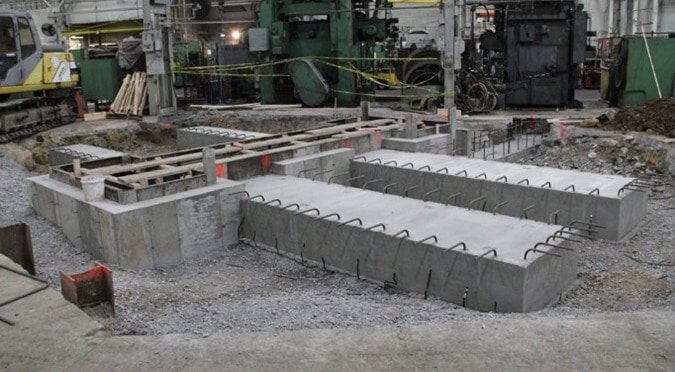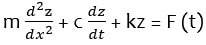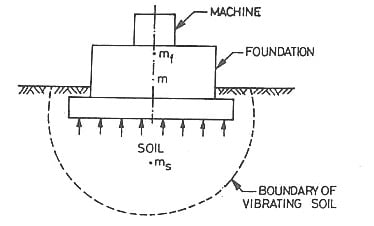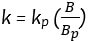# Vibration Analysis of Machine Foundations

Machine Foundation is subjected to dynamic loads. These loads develop the vibratory motions which will transmit into the soil below the foundation. The effect on soil caused by these vibrations is analyzed using principles of soil dynamics and theory of vibrations.

## Types of Vibrations in Machine Foundation

There are two types of vibrations in machine foundation:

• Free vibration
• Forced vibration### Free Vibration in Machine Foundation

Free vibrations occur without any external force and they occur under the influence of forces in the system itself. But to start free vibrations, initially an external force or natural disturbance is required. Free vibrations may be of two types as follows

• Damped vibrations
• Undamped vibrations

### Forced Vibration in Machine Foundation

Forced vibrations occur with continuous external forces on machine foundation. Let us say a damped system is subjected to exciting force F(t). The equation of motion can be written as## Vibration Analysis of Machine Foundation

To analyze the vibration theory of machine foundation we need to assume that the machine foundation has single degree of freedom. Normally machine foundation has 6 degree of freedom.

Let us say a machine foundation is rest on soil mass. Now the mass of machine and foundation acts downwards together and it is say mf which acts at the center of gravity of the system. The mass of soil which acts upwards is say ms. the elastic action of soil due to vibration of system is dependent of stiffness k. Resistance against motion is dependent of damping coefficient c.

So, these three mass, stiffness and damping coefficient are required to complete the analysis of machine foundation. Determination of above parameters is explained below.### Mass (m)

Whenever the machine vibrates, soil below the machine foundation also vibrates. The mass of soil which vibrates due to machine vibration is termed as in-phase soil mass. Therefore, total mass (m) is equal to

m = mf + ms

Where,

mf = mass of the foundation

ms = in-phase soil mass = it varies from 0 to mf

Total mass (m) varies from mf to 2mf.

### Stiffness (k)

The stiffness is dependent of type of soil below the foundation, embedment of foundation block and contact pressure distribution between soil and foundation. Stiffness is derived from the following methods.

## Laboratory Method of Vibration Analysis

In the laboratory, a tri axial test with vertical vibrations is performed and modulus of rigidity is obtained. From this young’s modulus is determined with the help of Poisson’s ratio.

Young’s modulus E = 2G(1+u)

Stiffness k = AE/L

Where E = young’s modulus

G = rigidity modulus

U = positions ratio

### Barkan’s Method

The stiffness can also be derived from the formula proposed by Barkan which is given below.Where A = area of contact

A plate load test is conducted in the field and determine the stiffness of soil as slope of the load-deformation curve.

For cohesive soils, Stiffness KFor cohesionless soils, Stiffness kWhere,

B = width of foundation

Bp = diameter of plate

### Resonance Test

By knowing the resonance frequency (fn), we can calculate the stiffness value. fncan be determined by placing vibrator of mass m on a steel plate supported on ground.Therefore, stiffness### Damping Constant (c)

When the vibration energy dissipates from the soil, damping occurs. The main reasons to develop damping is internal friction loss due to viscous effects and hysteresis, radiational losses due to propagation of waves through soil.

The damping constant c is obtained from the area of hysteresis loop of load deformation curve as followsWhere,= work lost in hysteresis

= total work done.

The damping constant varies from 0.01 to 0.1